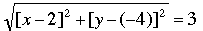SEARCH HOMEMath Central Quandaries & QueriesQuestion from lupe, a student: Consider the circle centered at (2,-4) with radius 3. Write the equation of this circle sketch its graph, and find the exact coordinates of its intercepts. x-intercept y-intercept using the quadratic equation?Hi Lupe,

You can tell if a point (x,y) in the plane is on the circle by finding the distance from (x,y) to (2,-4). If the distance is 3 units it's on the circle and if the distance is not 3 it's not on the circle. To say this using the distance formula I get, (x,y) is on the circle if and only if.

Square both sides and simplify to obtain the equation of the circle.

The x-intercepts are the x-coordinates of the points where the graph crosses the x-axis. Thus for these points the y-coordinate is zero. Thus, in the equation set y = 0 and solve for x. Similarly to find the y-intercepts, set x = 0 in the equation and solve for x.

On graph paper set up a coordinate system and use a compass to draw the circle with center (2,-4) and radius 3. Look at the points on the graph where is crosses the x- and y-coordinates. Do they agree with the intercepts you found?

Cheers,
PennyMath Central is supported by the University of Regina and The Pacific Institute for the Mathematical Sciences.